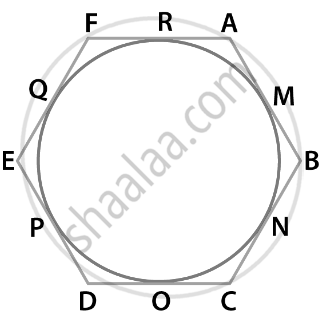# If a hexagon ABCDEF circumscribe a circle, prove that AB + CD + EF = BC + DE + FA. - Mathematics

Sum

If a hexagon ABCDEF circumscribe a circle, prove that AB + CD + EF = BC + DE + FA.

#### SolutionA Hexagon ABCDEF circumscribe a circle.

To prove: AB + CD + EF = BC + DE + FA

Proof: Tangents drawn from an external point to a circle are equal.

Hence, we have

AM = RA  ......Equation 1 [tangents from point A]

BM = BN ......Equation 2 [tangents from point B]

CO = NC ......Equation 3 [tangents from point C]

OD = DP ......Equation 4 [tangents from point D]

EQ = PE ......Equation 5 [tangents from point E]

QF = FR  ......Equation 6 [tangents from point F] [equation 1] + [equation 2] + [equation 3] + [equation 4] + [equation 5] + [equation 6]

AM + BM + CO + OD + EQ + QF = RA + BN + NC + DP + PE + FR

On rearranging, we get,

(AM + BM) + (CO + OD) + (EQ + QF) = (BN + NC) + (DP + PE) + (FR + RA)

AB + CD + EF = BC + DE + FA

Hence Proved!

Concept: Concept of Circle - Centre, Radius, Diameter, Arc, Sector, Chord, Segment, Semicircle, Circumference, Interior and Exterior, Concentric Circles
Is there an error in this question or solution?

#### APPEARS IN

NCERT Mathematics Exemplar Class 10
Chapter 9 Circles
Exercise 9.4 | Q 1 | Page 110
Share